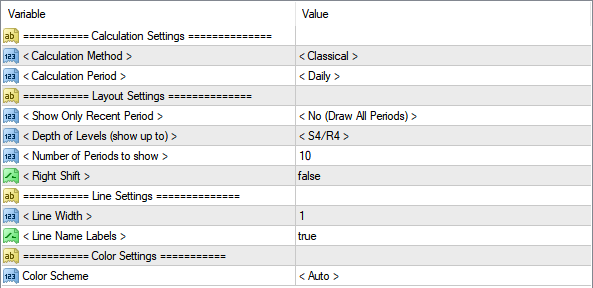FXSSI

# Pivot Points.All-in-oneFree

## 枢轴点的用法## 枢轴点图形的画法与公式

PP – 枢轴点；
S1-S4 – 支撑价位线；
R1-R4 – 阻力价位线；

### 标准枢轴点图形（Floor）

PP = (H + L + C) / 3
R1 = (2 * PP) – L
R2 = PP + RANGE
R3 = PP + RANGE * 2
R4 = PP + RANGE * 3
S1 = (2 * PP) – H
S2 = PP – RANGE
S3 = PP – RANGE * 2
S4 = PP – RANGE * 3

### 卡马利拉枢轴点（Camarilla）

R1 = C + RANGE * 1.1 / 12
R2 = C + RANGE * 1.1 / 6
R3 = C + RANGE * 1.1 / 4
R4 = C + RANGE * 1.1 / 2
S1 = C – RANGE * 1.1 / 12
S2 = C – RANGE * 1.1 / 6
S3 = C – RANGE * 1.1 / 4
S4 = C – RANGE * 1.1 / 2
PP = (S1 + R1) / 2

### 伍迪枢轴点（Woodie）计算方法

PP = (H + L + (C * 2)) / 4
R1 = (2 * PP) – L
R2 = PP + RANGE
R3 = R1 + RANGE
R4 = R3 + RANGE
S1 = (2 * PP) – H
S2 = PP – RANGE
S3 = S1 – RANGE
S4 = S3 – RANGE

### 斐波那契枢轴点（Fibonacci）

PP = (H + L + C) / 3
R1 = PP + (R x 0.382)
R2 = PP + (R x 0.618)
R3 = PP + (R x 1.000)
R4 = PP + (R x 1.618)
S1 = PP – (R x 0.382)
S2 = PP – (R x 0.618)
S3 = PP – (R x 1.000)
S4 = PP + (R x 1.618)

### 中心枢轴范围（CPR）

CPR枢轴点的计算公式如下：
TC = (Pivot – BC) + Pivot
Pivot = (High + Low + Close)/3
BC = (High + Low)/2

TC – 上边界线
Pivot – 中心线
BC – 下边界线

## 枢轴点技术图形的设置Calculation Method (计算方法) – 选择枢轴点技术图形的计算方法：标准方式（Classical）、卡马利拉（Camarilla）、伍迪（Woodie）、斐波那契（Fibonacci）。这4种计算方法的公式已在本文前面进行了说明。

Calculation Period (计算的K线周期) – 这里设置计算前一周期的高点、低点和收盘价的K线的周期，如日K线。

• 分时
• 日线
• 周线
• 月线

Number of Periods to show (显示的K线周期数)在枢轴点图形上显示的过去的K线周期数。如果此选项设置为“只显示当前周期Show only the current period”，则这项设置不会起作用。

Show Only Recent Period (只显示最近的周期) 显示一个或多个计算周期。

Depth of Levels (价位线的数量)  – 在枢轴点技术图形中，支撑线和阻力线的显示数量。

Right Shift (线条右延长) – 枢轴点图形中的线条向右延长直到边界。

Color Scheme (颜色设置) – 自动为枢轴点图形选用合适的颜色。也可以手动变更图形的背景颜色。

## 如何将技术指标Pivot Points.All-in-one添加到MT4/MT5

1. 点击本页上方的链接，下载技术指标Pivot Points.All-in-one 的.zip压缩包；
2. 将压缩包解压到MT4客户端安装位置的 MQL4/5/indicators 目录下；
3. 重新打开 MT4/5 客户端；
4. 在 MT4/5 的导航器内双击技术指标的名称；
5. 勾选”Allow DLL imports(允许导入动态链接库)” 前的方框，点击 “OK”；
6. 技术指标此时会自动显示在行情图中；
7. 按照使用习惯修改技术指标的设置。使用快捷键 CTRL+I，从列表中选择技术指标，然后点击 “Inputs(输入参数)” 标签。

```    index: 1x 0.024658918380737s
t_/pages/product: 1x 0.016395092010498s